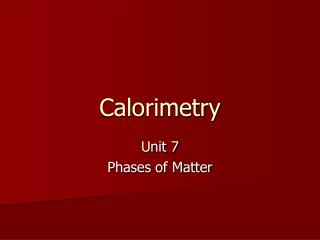DownloadDownload PresentationCalorimetry

# Calorimetry

Download Presentation## Calorimetry

- - - - - - - - - - - - - - - - - - - - - - - - - - - E N D - - - - - - - - - - - - - - - - - - - - - - - - - - -
##### Presentation Transcript

1. Calorimetry Unit 7 Phases of Matter

2. Calorimetry • Allows us to calculate the amount of energy required to heat up a substance or to make a substance change states. • Molar Heat of Fusion— The heat absorbed by one mole of a substance when changing from a solid to a liquid. • For water, it = 6.0 kiloJoules/mole • Heat of solidification is opposite of heat of fusion (heat is released).

3. Molar Heat of Vaporization— The heat absorbed by one mole of a substance when changing from a liquid to a gas. • For water, it = 40.7 kiloJoules/mole. • Heat of condensation is the opposite of heat of vaporization (heat is released) Heat Required For a Phase Change Heat Absorbed or Released = q q = (moles) x (Molar Heat Fusion/Vaporization)

4. Calculating Heat Required To Change State • Example #1: How much heat is needed to melt 56.0 grams of ice into liquid (the molar heat of fusion for ice is 6.0 kJ/mol)? • 56.0 g 1 mole H2O 6.0 kJ = 18.0 g 1 mole • = 18.7 kJ will be absorbed

5. Example #2 • How much heat energy will be released when 200grams steam condenses back to a liquid water? • Molar Heat condensation = 40.7kJ/mol q = (moles) x (Molar Heat condensation) 200gram 1 mole 40.7 kJ 18gram 1 mole = 452 kJ released

6. Heating a Substance with No Phase Change • Specific Heat Capacity--The amount of energy required to raise one gram of a substance one degree Celcius. Water’s Specific Heat (as a liquid) = 4.184 Joules/gram oC

7. Energy to Change Temperature q = (mass) ( C ) ( T ) Change in Temperature Tfinal – Tinitial In OCelcius Heat Measured in Joules Specific Heat Capacity Mass In grams

8. Example #3 • How much energy is needed to heat 80grams of water from 10oC to 55oC? q = m C T = m C (Tfinal – Tinitial ) q = (80grams) ( 4.184 J/goC) (55oC – 10oC) q = 15062 joules divide by 1000 to get kilojoules 15062 J 1 kJ = 1000J 15.06 kJ absorbed

9. This problem requires two steps. Since water is solid ice at 0oC, we need to melt the ice and then heat it up to 50oC. • Example #4 -How much energy is needed to change 150grams of ice from 0oC to 50oC? Step 1 – Calculate heat required to melt 150grams ice 150g 1 mole 6.0 kJ = 50 kJ 18grams 1 mole Step 2 - Calculate heat required to heat liquid water from 0oC to 50oC q = mC T = (150g)(4.184 J/goC)(50oC) = 31380 J  convert to kJ = 31.38kJ *Add both heat values together for your final answer 50 kJ + 31.38kJ = 81.38 kJ heat absorbed.

10. Multiple Step Calorimetry Problems Use q = Moles x Molar Heat vap/fus Gas Heats Vaporization Liquid Heats Solid Heats melting Use q = mC T * Add each individual energies (in kJ) together for total